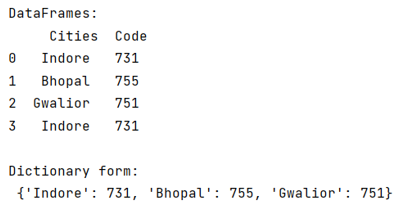# How to create a dictionary of two Pandas DataFrames columns?

Given two Pandas DataFrames, we have to create a dictionary.
Submitted by Pranit Sharma, on June 07, 2022

## Pandas DataFrames Columns

Columns are the different fields that contain their particular values when we create a DataFrame. We can perform certain operations on both rows & column values.

## Create a dictionary of two Pandas DataFrames columns

To create a dictionary of two pandas DataFrame columns - we will use zip() method, it will combine the values of both columns then we will convert the result into a dictionary.

To apply this method to specific columns, we need to define the specific columns at time of function calling.

Note

To work with pandas, we need to import pandas package first, below is the syntax:

```import pandas as pd
```

Let us understand with the help of an example,

## Python program to create a dictionary of two Pandas DataFrames columns

```# Importing pandas package
import pandas as pd

# Creating a DataFrame
df = pd.DataFrame({
'Cities':['Indore','Bhopal','Gwalior','Indore'],
'Code':[731,755,751,731]
})

# Display DataFrames
print("DataFrames:\n",df,"\n")

# Using Zip method
ans = zip(df.Cities,df.Code)

# Converting it into list
ans = list(ans)

# Converting it into dictionary
ans = dict(ans)

# Display ans
print("Result of apply:\n",ans,"\n")
```

## Output

The output of the above program is: